• 基于 MATLABPID 控制器设计 基于 MATLABPID 控制器设计PID 控制简介 PID 控制是最早发展起来的经典控制策略 , 是用于过程控制最有效的 策略之一由于其原理简单技术成在实际应用中较易于整定 , 在工业 控制...
• 基于MATLABPID控制器设计.rar 基于MATLABPID控制器设计.rar 基于MATLABPID控制器设计.rar基于MATLABPID控制器设计.rar
• MATLAB 论文 -- 基于控制系统的 PID 调节 基于 MATLABPID 控制器 摘要本论文主要研究 PID 控制器 PID 控制是迄今为止最通用的控制方法 大多数反馈回路用该方法或其较小的变形来控制 PID 控制器亦称调节器及 其...
• 基于 MATLABPID 控制器设计PID 控制简介 PID 控制是最早发展起来的经典控制策略, 是用于过程控制最有效的策 略之一由于其原理简单技术成在实际应用中较易于整定, 在工业控 制中得到了广泛的应用它最大的优点...
• 023 文章编号:1000 －2243( 2011) 06 －0898 －06 运用 Matlab 实现 PID 控制器设计 郑剑翔 ( 福州大学现代教育技术中心，福建 福州 350108) 摘要: 传统的 PID 控制器设计中的参数调试耗时费力且调节不当还可能...
第 39 卷 第 6 期 2011 年 12 月 福州大学学报( 自然科学版) Journal of Fuzhou University( Natural Science Edition) Vol． 39 No． 6 Dec． 2011 DOI: CNKI:35 －1117/N． 20111220． 0957． 023 文章编号:1000 －2243( 2011) 06 －0898 －06 运用 Matlab 实现 PID 控制器的设计 郑剑翔 ( 福州大学现代教育技术中心，福建 福州 350108) 摘要: 传统的 PID 控制器设计中的参数调试耗时费力且调节不当还可能损坏机器． 使用 2009b 版 Matlab 软件新增的 PID 控制模块，可方便快捷地完成PID 调试工作． 以设计液压式旋切机控制器为例，分析使用 Matlab 的PID 控制模块、定点工具以及嵌入式实时代码工具完成 PID 控制器的设计，包括对象建模、离散化和定点运算处理等工作． 关键词: PID 控制; Matlab; 无卡轴旋切机; 液压 中图分类号: TP271． 2 文献标识码: A An implementation method for PID controller design by Matlab ZHENG Jian － xiang ( Modern Education Technology Center of Fuzhou University，Fuzhou，Fujian 350108，China) Abstract: Parameters adjustment is a complicated and time － consuming work in traditional PID con-troller design usually，inappropriate adjustment can cause hardware damage． By using newly added PID controller module in 2009b version of Matlab software，PID adjustments will become more conven-ience and faster． It can become easily to do PID controller design，including user’s plant modling，discrete － time implementation and fixed － point scaling in the design． Using a controller design proce-dure of hydraulic veneer lathe as example，this paper introduces how to use PID control module，fixed － piont toolbox，and embedded real － time coder of Matlab to design a PID controller easily． Keywords: PID control; Matlab; veneer peeling lathe; hydraulic pressure 0 引言 调试 PID 控制器需要确定三个参数值: 比例、积分和微分的增益． 要安全系统地找到能保证控制系统最佳运行的一组参数是一件复杂的工作［1］． 用传统的方法调试，
展开全文• 基 于 M A T L A B 的 P I D 控 制 设 计 一 PID 控制简介 PID 控制是最早发展起来的经典控制策略 , 是用于过程控制最有效的策略之 一由于其原理简单技术成在实际应用中较易于整定 , 在工业控制中得到了广 泛的...
• ## MATLAB仿真PID控制器与模糊控制器

千次阅读 多人点赞 2019-03-04 16:06:10
摘要：使用matlab编写PID控制器与模糊控制器，并对原理进行解析。 背景： 小白自学。 基础：1、视频“自动控制原理”，1-3章，柠檬大学，点此链接 2、《基于MATLAB的系统分析与设计——模糊系统》，楼顺天等，...
摘要：使用matlab编写PID控制器与模糊控制器，并对原理进行解析。

背景： 小白自学。

基础：1、视频“自动控制原理”，1-3章，柠檬大学，点此链接

2、《基于MATLAB的系统分析与设计——模糊系统》，楼顺天等，西安电子科技大学出版社，1-80页。

3、视频“单片机STM32——PID算法讲解”，1-6节，ArgonGhost，哔哩哔哩，点此链接。

4、视频“第9章线性系统的状态空间分析与综合（1）”，第一节，张超，哔哩哔哩，点此链接。

目录

一、控制案例

二、重要概念说明

2.1 PID控制原理

2.1.1 位置式PID

2.1.2 增量式PID

2.2 状态空间标准式

2.3 龙格-库塔法

一、控制案例

在此通过一个典型案例编写PID控制器和模糊控制器代码，并进行注释。（例子为《基于MATLAB的系统分析与设计——模糊系统》例3.8）

例：典型二阶系统的模糊控制与 传统PID控制的性能比较。通常的工业过程可以等效成二阶系统加上一些典型的非线性环境，如死区、饱和、纯延迟等，这里假设系统为

（1）

控制执行结构具有0.07的死区和0.7的饱和区，取样时间间隔T=0.01。

解：在PID仿真中，经过仔细选择，取，，。在模糊控制仿真中，，，，，模糊控制器输出为

（2）

其中积分项用于消除控制系统的稳态误差。

MATLAB程序中，Nd用于表示系统的纯延迟（），umin用于表示控制的死区电平，umax用于表示饱和电平。当Nd=0时，表示系统不存在纯延迟。MATLAB程序如下：

% -----------------------------------------
% 典型二阶系统的模糊控制与传统PID控制的性能比较
% -----------------------------------------
num=20;
den=[1.6,4.4,1];
[a1,b,c,d]=tf2ss(num,den);           %将传递函数转换为状态空间，
x=[0;0];                             %有两个输入所以状态向量为二维
T=0.01;h=T;
umin=0.07;umax=0.7;                  %死区和饱和区
td=0.02;Nd=td/T;
N=500;R=1.5*ones(1,N);               %稳态为1.5，存入R一维矩阵
% -------------
% PID 控制
% -------------
e=0;de=0;ie=0;                       %初始误差、误差微分、误差积分
kp=5;ki=0.1;kd=0.001;                %PID控制器的三个参数
for k=1:N                            %重复1~500
uu1(1,k)=-(kp*e+ki*de+kd*ie);    %计算本次PID控制器的输出
if k<=Nd                         %延迟调整
u=0;
else
u=uu1(1,k-Nd);
end
if abs(u) <= umin                %死区和饱和区调整
u=0;
elseif abs(u) > umax
u=sign(u) * umax;
end

k0=a1*x+b*u;                     %利用龙格-库塔法进行系统仿真，
k1=a1*(x+h*k0/2)+b*u;
k2=a1*(x+h*k1/2)+b*u;
k3=a1*(x+h*k2)+b*u;
x=x+(k0+2*k1+2*k2+k3)*h/6;
y=c*x+d*u;

e1=e;                            %计算误差、微分和积分
e=y(1,1)-R(1,k);
de=(e-e1)/T;
ie=e*T+ie;
yy1(1,k)=y;                      %将本次系统输出存入yy1矩阵
end
% -------------
% 模糊控制
% -------------
a=newfis('Simple');
rr =[5 5 4 4 3                            %以下为计算模糊规则
5 4 4 3 3
4 4 3 3 2
4 3 3 2 2
3 3 2 2 1];
r1=zeros(prod(size(rr)),3);k=1;
for i=1:size(rr,1)
for j=1:size(rr,2)
r1(k,:)=[i,j,rr(i,j)];
k=k+1;
end
end
[r,s]=size(r1);
r2=ones(r,2);
rulelist=[r1,r2];                    %计算模糊规则结束
e=0;de=0;ie=0;                       %初始化误差、误差微分、误差积分
x=[0;0];                             %初始化状态向量
ke=60;kd=2.5;ki=0.01;ku=0.8;         %模糊控制器参数
for k=1:N                            %1~500
e1=ke*e;                         %输入变量变换至论域
de1=kd*de;
if e1>=6                         %误差边界调整
e1=6;
elseif e1<=-6
e1=-6;
end
if de1>=6                        %误差微分边界调整
de1=6;
elseif de1<=-6
de1=-6;
end
in=[e1 de1];                     %输入向量
uu(1,k)=ku*evalfis(in,a)-ki*ie;  %根据式2计算模糊控制器输出
if k<=Nd                         %延迟调整
u=0;
else
u=uu(1,k-Nd);
end
if abs(u)<=umin                  %死区、饱和区调整
u=0;
elseif abs(u)>umax
u=sign(u)*umax;
end

k0=a1*x+b*u;                     %利用龙格-库塔法进行系统仿真
k1=a1*(x+h*k0/2)+b*u;
k2=a1*(x+h*k1/2)+b*u;
k3=a1*(x+h*k2)+b*u;
x=x+(k0+2*k1+2*k2+k3)*h/6;
y=c*x+d*u;

e1=e;                            %计算误差、微分和积分
e=y-R(1,k);
de=(e-e1)/T;
ie=ie+e*T;
yy(1,k)=y;                       %将本次系统输出存入yy矩阵
end
% -------------
% 画图比较
% -------------
kk=[1:N]*T;
figure(1);
plot(kk,R,'k',kk,yy1,'b',kk,yy,'r'); %将PID控制和模糊控制同时显示
xlabel('时间（0.01秒）');
ylabel('输出');
gtext('模糊控制');gtext('PID控制');


运行，输出如下：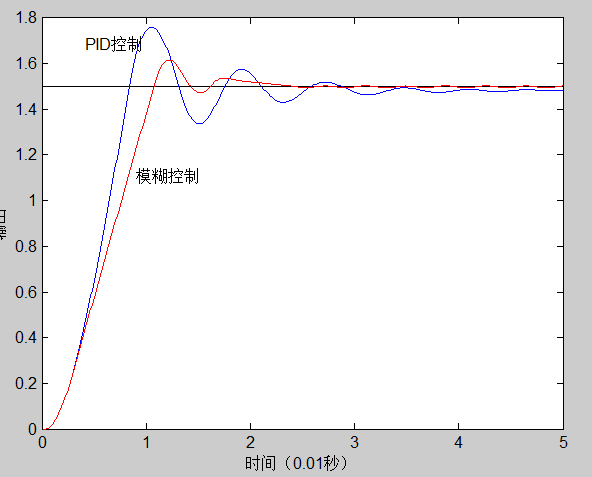二、重要概念说明

2.1 PID控制原理

PID控制分为位置式控制和增量式控制，大多数情况下为位置式PID控制。

2.1.1 位置式PID

基本公式：                （3）

即：                                            （4）

其中                                                                                                                          （5）

（6）

其中：为比例系数，为k时刻的误差，为采样周期（计算周期，控制周期），为积分常数（积分时间），为微分常数

采样周期：单片机不能不停计算输出，因为可能上一次的计算输出还没有加到控制对象上，需要根据实际系统延迟。

积分常数：和共同作用的时间，越大，减弱输出信号；越小，增加输出信号；相当于历史误差的惩罚权值，人为给定。

例，早上第一次温度调控，室温为20度，目标为100度，当从20度第一次到100度时，由于达到目标值输出为0，但积分项考虑历史数据累积了大量误差，所以仍然会有输出，产生超调。有些算法为了避免超调，在第一次到达目标值时将积分常数选得非常大，减弱积分项的作用，这种方法为积分分离。积分项用于在比例项达标时控制（此时比例项无输出），即在到达目标之后才能体现积分项的作用。

微分常数：和共同作用的时间，采样周期越大，相同误差下误差变化率越小（影响）。相当于误差变化的惩罚权值，人为给定。

2.1.2 增量式PID

位置式PID的输出量为直接控制量； 增量式PID的输出为控制变化量，即实际输出为当前控制量加上控制变化量，公式如下：

2.2 状态空间标准式

在说明状态空间标准式之前，说明下本例子总体控制模型，如下：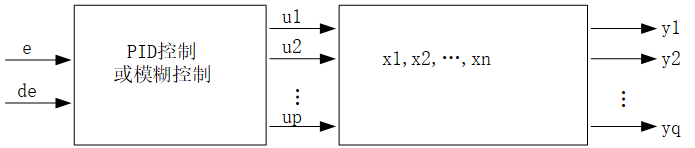线性系统状态空间描述：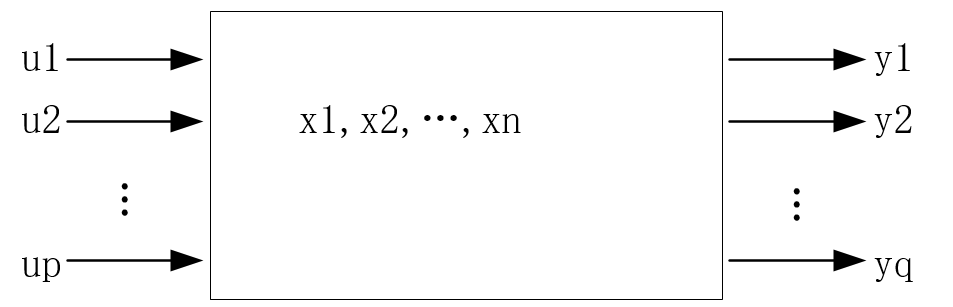输入向量：

输出向量：

系统内部状态向量：，中间某一时刻的状态。

状态空间标准式：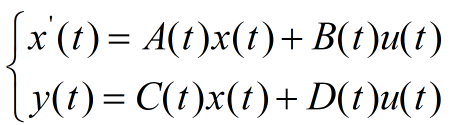（7）

其中，x为n维向量，u为p维向量，y为q维向量，A为nxn矩阵，B为nxq矩阵，C为qxn矩阵，D为qxp矩阵。

本文重点！：在此案例中u(t)为PID控制器或模糊控制器输出量。整体过程为：首先根据当前误差和误差变化输入到PID控制器或模糊控制器，输出为u(t)，然后根据龙格-库塔法对状态空间标准式（即7式）求出输出y。

2.3 龙格-库塔法

该方法不用求解微分方程直接计算下一个点的值，以下摘百度百科。

在各种龙格－库塔法当中有一个方法十分常用，以至于经常被称为“RK4”或者就是“龙格－库塔法”。该方法主要是在已知方程导数和初值信息，利用计算机仿真时应用，省去求解微分方程的复杂过程。

令初值问题表述如下。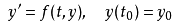则，对于该问题的RK4由如下方程给出：这样，下一个值（yn+1）由现在的值（yn）加上时间间隔（h）和一个估算的斜率的乘积所决定。该斜率是以下斜率的加权平均：

k1是时间段开始时的斜率；

k2是时间段中点的斜率，通过欧拉法采用斜率k1来决定y在点tn+h/2的值；

k3也是中点的斜率，但是这次采用斜率k2决定y值；

k4是时间段终点的斜率，其y值用k3决定。

当四个斜率取平均时，中点的斜率有更大的权值：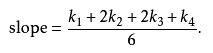RK4法是四阶方法，也就是说每步的误差是h阶，而总积累误差为h阶。

注意上述公式对于标量或者向量函数（y可以是向量）都适用。

展开全文• 这是基于matlab的pid控制方式，可以用matlab设计pid控制器，更加简洁优化
• 基于MATLAB模糊自适应PID 控制器设计The fuzzy PID controller and‘its simulation Abstract：This paper discusses the design of fuzzy PID controller and he method of realization using MATLAB software．...
基于MATLAB模糊自适应PID 控制器的设计The fuzzy PID controller and‘its simulation Abstract：This paper discusses the design of fuzzy PID controller and he method of realization using MATLAB software．The result of simulation in MATLAB／Simulink indicates that the controlle r evidently improves the dynamic property of control system．The controlle r is easily realized and applied in engineering．First, let's take a look at how the PID controller works in variable (e) represents the tracking error, the difference between the desired input value (R) and the actual output (Y). This error signal (e) will be sent to the PID controller, and the controller computes both the derivative and the integral of this error signal. The signal (u) just past the controller is now equal to the proportional gain (Kp) times the magnitude of the error plus the integral gain (Ki) times the integral of the error plus the derivative gain (Kd) times the derivative
展开全文• 基于MATLAB的模糊PID控制器设计，使用简单，易上手。
• 基于MATLABPID_控制器设计，较为详细的一个设计过程
• matlab开发-优化PID控制器设计接口。optimpid是一个基于matlab的接口，可以用来优化PID参数。
• 成绩 课程设计报告 题 目 PID控制器的应用 课 程 名 称 控制系统仿真 院 部 名 称 机电工程学院 专 业 自动化 班 级 09自动化 学 生 姓 名 学 号 课程设计地点 C208 课程设计学时 1 周 指 导 教 师 金陵科技学院教务...
• PID 控制器设计和调整与 MATLAB 和模拟
• PID控制器与状态反馈控制器MATLAB教学实例设计(投稿版）.pdf
• 基于MATLAB仿真的PID控制器设计毕业设计 基于 MATLAB 仿真的 PID 控制器设计 摘 要 本论文以温度控制系统为研究对象设计一个 PID 控制器。PID 控制是迄今为止最通用的控制方法， 大多数反馈回路用该方法或其较小的...基于MATLAB仿真的PID控制器设计毕业设计 基于 MATLAB 仿真的 PID 控制器设计 摘 要 本论文以温度控制系统为研究对象设计一个 PID 控制器。PID 控制是迄今为止最通用的控制方法， 大多数反馈回路用该方法或其较小的变形来控制。PID 控制器(亦称调节器)及其改进型因此成为工 业过程控制中最常见的控制器 (至今在全世界过程控制中用的 84%仍是纯 PID 调节器，若改进型包含 在内则超过 90%)。在 PID 控制器的设计中，参数整定是最为重要的,随着计算机技术的迅速发展，对 PID 参数的整定大多借助于一些先进的软件，例如目前得到广泛应用的 MATLAB 仿真系统。本设计就是 借助此软件主要运用 Relay-feedback 法，线上综合法和系统辨识法来研究 PID 控制器的设计方法，设 计一个温控系统的 PID 控制器，并通过 MATLAB 中的虚拟示波器观察系统完善后在阶跃信号下的输 出波形。 关键词关键词： PID 参数整定 ；PID 控制器 ；MATLAB 仿真；冷却机； Design of PID Controller based on MATLAB Abstract This paper regards temperature control system as the research object to design a pid controller. Pid control is the most common control up until now; the great majority feedback loop is controlled by this or its small deation. Pid controller (claim regulator also) and its second generation so become the most common controllers in the industry process control (so far, about 84% of the controller being used is the pure pid controller, it’ll exceed 90% if the second generation included). Pid parameter setting is most important in pid controller designing, and with the rapid development of the computer technology, it mostly recurs to some advanced software, for example, mat lab simulation software widely used now. this design is to apply that soft mainly use Relay feedback law and synthetic on the line to study pid controller design , design a pid controller of temperature control system and observe the output wave while step signal through virtual oscilloscope after system completed. Keywords: PID parameter setting ；PID controller； MATLAB simulation；cooling machine 目 录 摘 要.I ABSTRACTII 第一章 绪 论1 1.1 课题来源及 PID 控制简介.1 1.1.1 课题的来源和意义 1 1.1.2 PID 控制简介.1 1.2 国内外研究现状及 MATLAB 简介3 第二章 控制系统及 PID 调节5 2.1 控制系统构成5 2.2 PID 控制5 2.2.1 比例、积分、微分 5 2.2.2 Ｐ、Ｉ、Ｄ控制 7 第三章 系统辨识9 3.1 系统辨识.9 3.2 系统特性图.10 3.3 系统辨识方法11 第四章 PID 最佳调整法与系统仿真14 4.1 PID 参数整定法概述.14 4.2 针对无转移函数的 PID 调整法15 4.2.1 RELAY FEEDBACK 调整法15 4.2.2 RELAY FEEDBACK 在计算机做仿真.15 4.2.3 在线调整法 19 4.2.4 在线调整法在计算机做仿真 20 4.3 针对有转移函数的 PID 调整方法.23 4.3.1 系统辨识法 24 4.3.2 波德图法及根轨迹法 27 第五章 油冷却机系统的 PID 控制器设计28 5.1 油冷却机系统28 5.1.1 油冷却机 28 5.1.2 感测与转换器 29 5.1.3 控制组件 30 5.2 油冷却机系统之系统辨识.31 5.3 油冷却机系统的 PID 参数整定33 结 论40 致 谢.41 参考文献.42 第一章 绪 论 1.1 课题来源及 PID 控制简介 1.1.1 课题的来源和意义 任何闭环的控制系统都有它固有的特性，可以有很多种数学形式来描述它， 如微分方程、传递函数、状态空间方程等。但这样的系统如果不做任何的系统 改造很难达到最佳的控制效果，比如快速性稳定性准确性等。为了达到最佳的 控制效果，我们在闭环系统的中间加入 PID 控制器并通过调整 PID 参数来改造 系统的结构特性，使其达到理想的控制效果。 1.1.2 PID 控制简介 当今的自动控制技术都是基于反馈的概念。反馈理论的要素包括三个部分： 测量、比较和执行。测量关心的变量，与期望值相比较，用这个误差纠正调节 控制系统的响应。 这个理论和应用自动控制的关键是，做出正确的测量和比较后，如何才能 更好地纠正系统，PID (比例 - 积分 - 微分)控制器作为最早实用化的控制 器已有 50 多年历史，现在仍然是应用最广泛的工业控制器。 PID 控制器简 单易懂，使用中不需精确的系统模型等先决条件，因而成为应用最为广泛的控 制器。 PID 控制器由比例单元( P ) 、积分单元( I )和微分单元( D )组成。 其输入 e (t) 与输出 u (t) 的关系为公式(1-1) 公式(1-1) 因此它的传递函数为公式(1-2) 公式(1-2) 比例调节作用：是按比例反应系统的偏差,系统一旦出现了偏差,比例调节立 即产生调节作用用以减少偏差。比例作用大,可以加快调节,减少误差,但是过大 的比例,使系统的稳定性下降,甚至造成系统的不稳定。 积分调节作用：是使系统消除稳态误差,提高无差度。因为有误差,积分调节 就进行,直至无差,积分调节停止,积分调节输出一个常值。积分作用的强弱取决 与积分时间常数 Ti，Ti 越小,积分作用就
展开全文• 本文主要研究的课题是：炉温系统的PID控制器设计研究 ，并且在MATLAB的大环境下进行模拟仿真。 (1)第一章 介绍课题的研究背景、意义以及发展现状。 (2)第二章 建立炉温系统数学模型 (3)第三章 常规PID控制和对常规...
• 模糊PID控制器设计MATLAB仿真 作者:李健, 王冬青, 王丽美 作者单位:青岛大学自动化工程学院,山东,青岛,266071 刊名: 工业控制计算机 英文刊名:INDUSTRIAL CONTROL COMPUTER 年,卷(期:2011,24(5 参考文献(4条 1....
• 本代码主要利用MATLAB工具进行MATLAB——基于粒子群算法的PID控制器优化设计的仿真
• 比较详细的阐述了模糊控制的原理，并给出了模糊PID仿真实例，与常规PID控制进行了比较
• 主要介绍了模糊PID控制器设计及其MATLAB仿真。
• 基于MATLAB的模糊PID控制器设计及其实现 沈 阳 化 工 大 学本 科 毕 业 论 文题 目： 基于 MATLAB 的模糊 PID 控制器的设计及其实现_ 院 系： 信息工程学院_____________ __ 专 业： 电气工程及其自动化__________...
• CMAC控制器设计与仿真：在小脑神经网络（CMAC）与PID 并行控制的基础上，提出了一种新型的CMAC 控制器，即 FCMAC 控制器
• Matlab 仿真——直流电机速度控制（3）PID控制器设计 上一节我们知道了我们的开环响应并不能满足设计需求，这一节我们通过一个PID控制器使我们的系统满足设计需求。 这里把设计需求和系统转换方程粘贴在这里： 设计......

# matlab设计pid控制器matlab 订阅